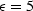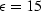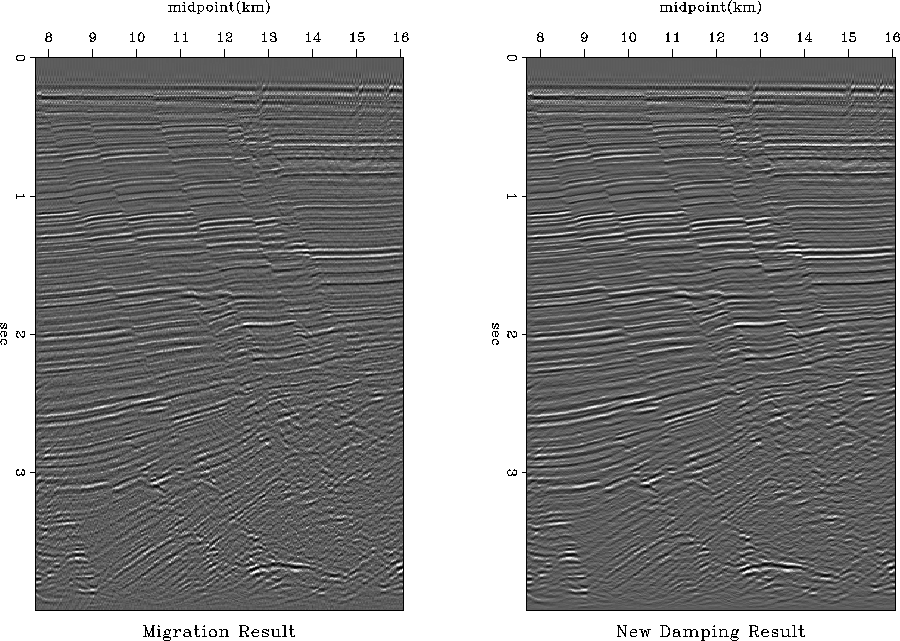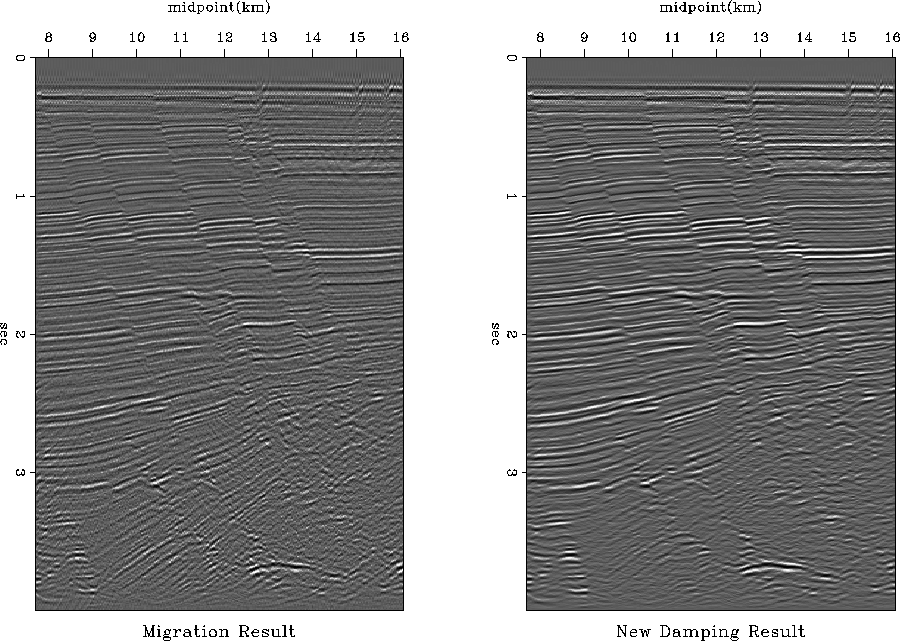Next: Angle results Up: Numerical Examples Previous: Numerical Examples

## The new R

Including causality and viscosity in the definition of R=ikz (equation 12) it is possible to preserve the energy content for any arbitraryvalue.

Figure 7 presents the modeling-migration results, using the model of synthetic data set in Figure 6, with equations (2) and (12) for two different values of.Figure 8 shows the respective frequency spectrum.mod3
Figure 6
Synthetic data setmod3_comp
Figure 7
Comparison between modeling-migration using model on Figure 6 using R definition as in equation (2), left, and equation (12), right. The top figures have a damping factor, the bottom figures have a damping factor ofmod3_freq
Figure 8
Comparison between the frequency spectrum of the modeling-migration results on Figure 7. The top figures have a damping factor,the bottom figures have a damping factor ofWe can assure the stability of the R definition presented in equation (12) based on the results of Figures 7 and 8; besides, this R definition is valid for all values ofand kx.

We also perform the phase-shift migration over a real data set, we present the results on Figures 9 and 10. These results show a comparison between phase-shift migration without considering the damping factor [R definition in equation (1)] and considering the damping factor [R definition in equation (12)].out1
Figure 9
Data Comparison. Left the migration result without considering damping. Right the migration result using the new R without2
Figure 10
Data Comparison. Left the migration result without considering damping. Right the migration result using the new R withThe image that we obtain with the damping factor is much cleaner than the image without the damping factor; therefore, the events definition and the faults are more clear.Next: Angle results Up: Numerical Examples Previous: Numerical Examples
Stanford Exploration Project
4/29/2001